September 16, 2020

## Announcements

• No assignments due this Sunday.
• Data Project
• Proposals are due October 25th (tentatively).
• Projects are to be done individually or in pairs.
• Pick any public data set to analyze. The goal is for you to do either a linear regression (preferred), null hypothesis test, ANOVA, or chi-squared test.
• Need to have at least three variables, typically two quantitative variables and one qualitative variable.
• Information, including a template and example proposal, are located here: https://fall2020.data606.net/assignments/project/

## Presentations

• 2.5 - Douglas Barley
• 2.11 - Diego Correa
• 2.27 - Stefano Biguzzi

## Coin Tosses Revisited

coins <- sample(c(-1,1), 100, replace=TRUE)
plot(1:length(coins), cumsum(coins), type='l')
abline(h=0)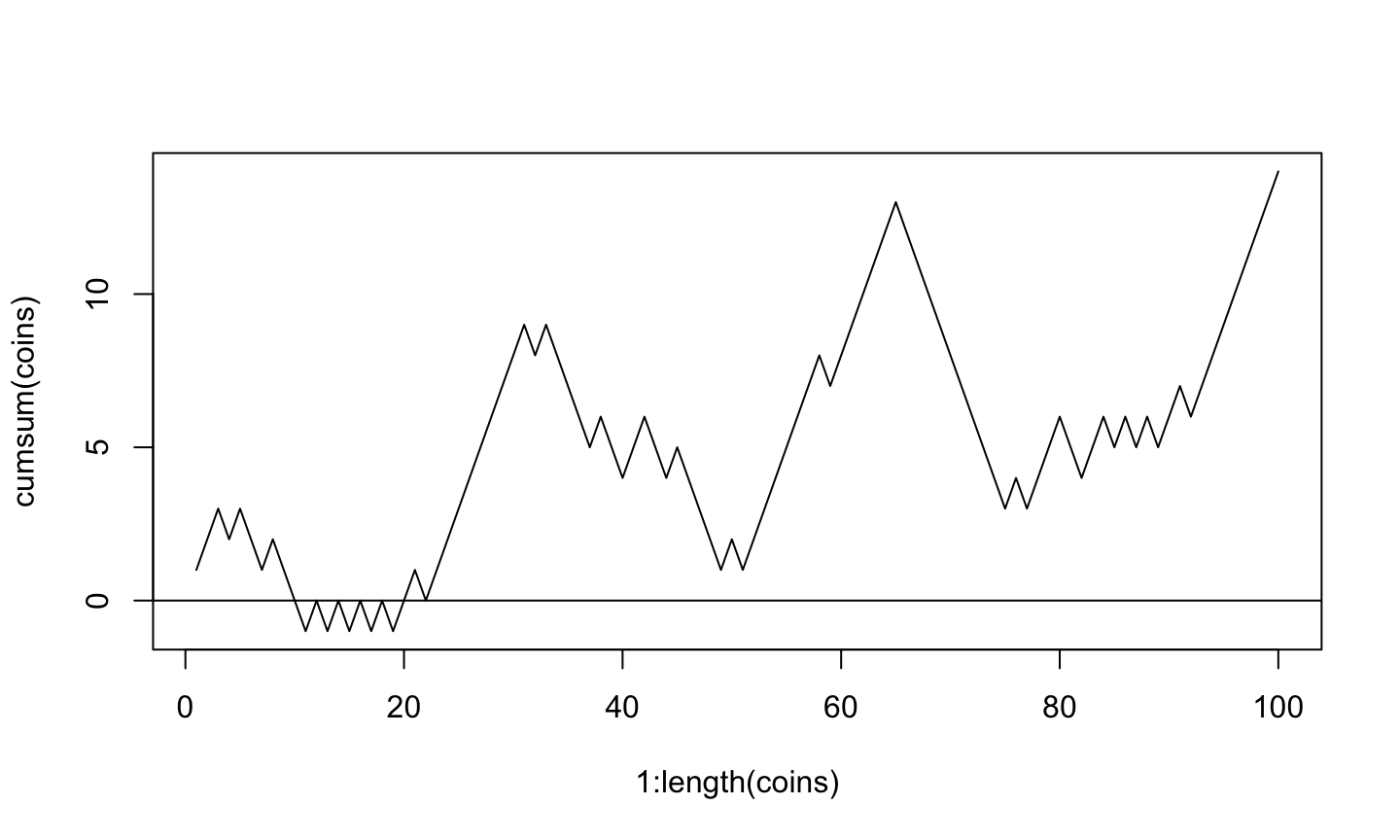cumsum(coins)[length(coins)]
##  14

## Many Random Samples

samples <- rep(NA, 1000)
for(i in seq_along(samples)) {
coins <- sample(c(-1,1), 100, replace=TRUE)
samples[i] <- cumsum(coins)[length(coins)]
}
head(samples)
##   0  8 -4 -6  2 -2

## Histogram of Many Random Samples

hist(samples)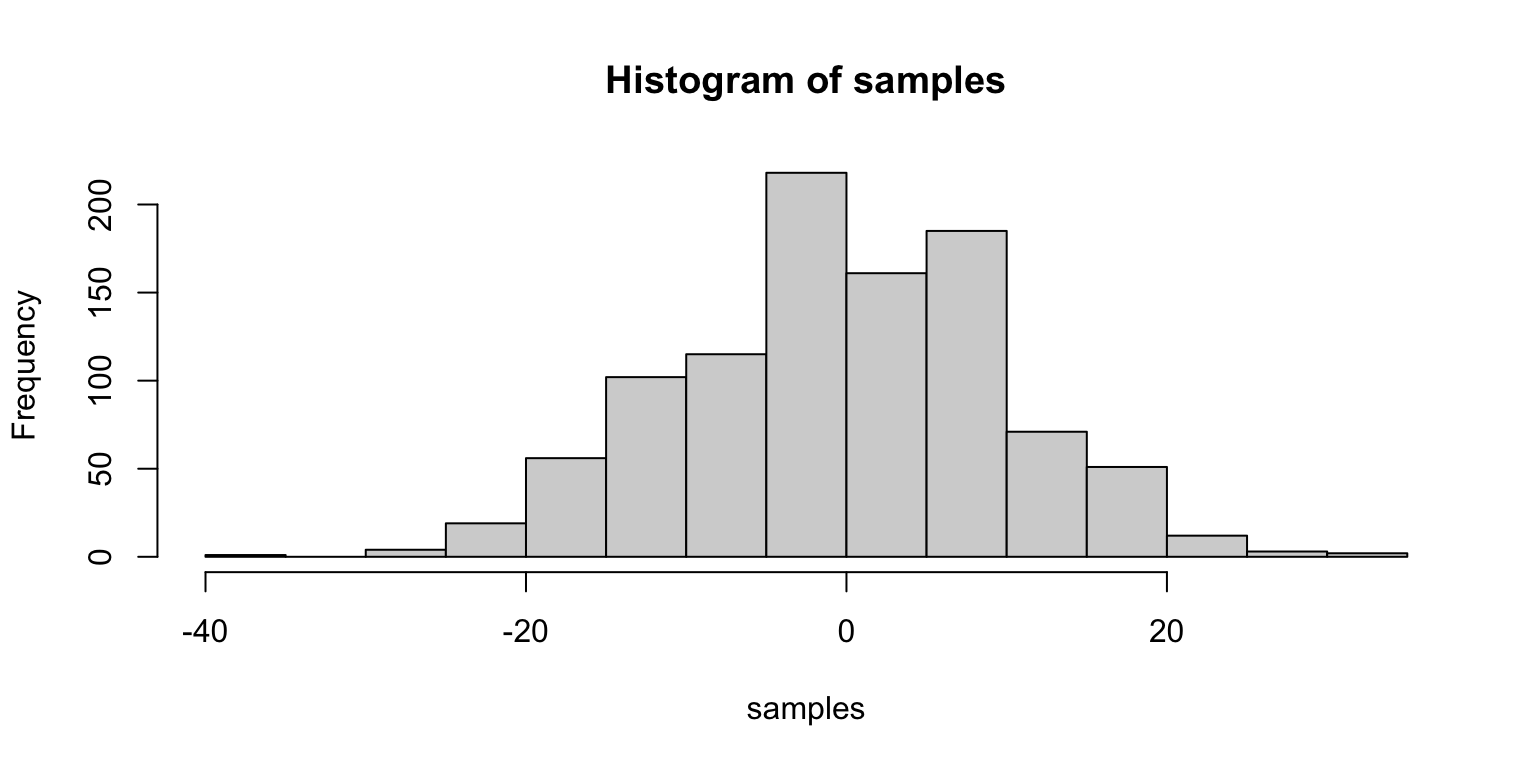## Properties of Distribution

(m.sam <- mean(samples))
##  0.244
(s.sam <- sd(samples))
##  10.05552

## Properties of Distribution (cont.)

within1sd <- samples[samples >= m.sam - s.sam & samples <= m.sam + s.sam]
length(within1sd) / length(samples)
##  0.679
within2sd <- samples[samples >= m.sam - 2 * s.sam & samples <= m.sam + 2* s.sam]
length(within2sd) / length(samples)
##  0.959
within3sd <- samples[samples >= m.sam - 3 * s.sam & samples <= m.sam + 3 * s.sam]
length(within3sd) / length(samples)
##  0.997

## Standard Normal Distribution

$f\left( x|\mu ,\sigma \right) =\frac { 1 }{ \sigma \sqrt { 2\pi } } { e }^{ -\frac { { \left( x-\mu \right) }^{ 2 } }{ { 2\sigma }^{ 2 } } }$

x <- seq(-4,4,length=200); y <- dnorm(x,mean=0, sd=1)
plot(x, y, type = "l", lwd = 2, xlim = c(-3.5,3.5), ylab='', xlab='z-score', yaxt='n')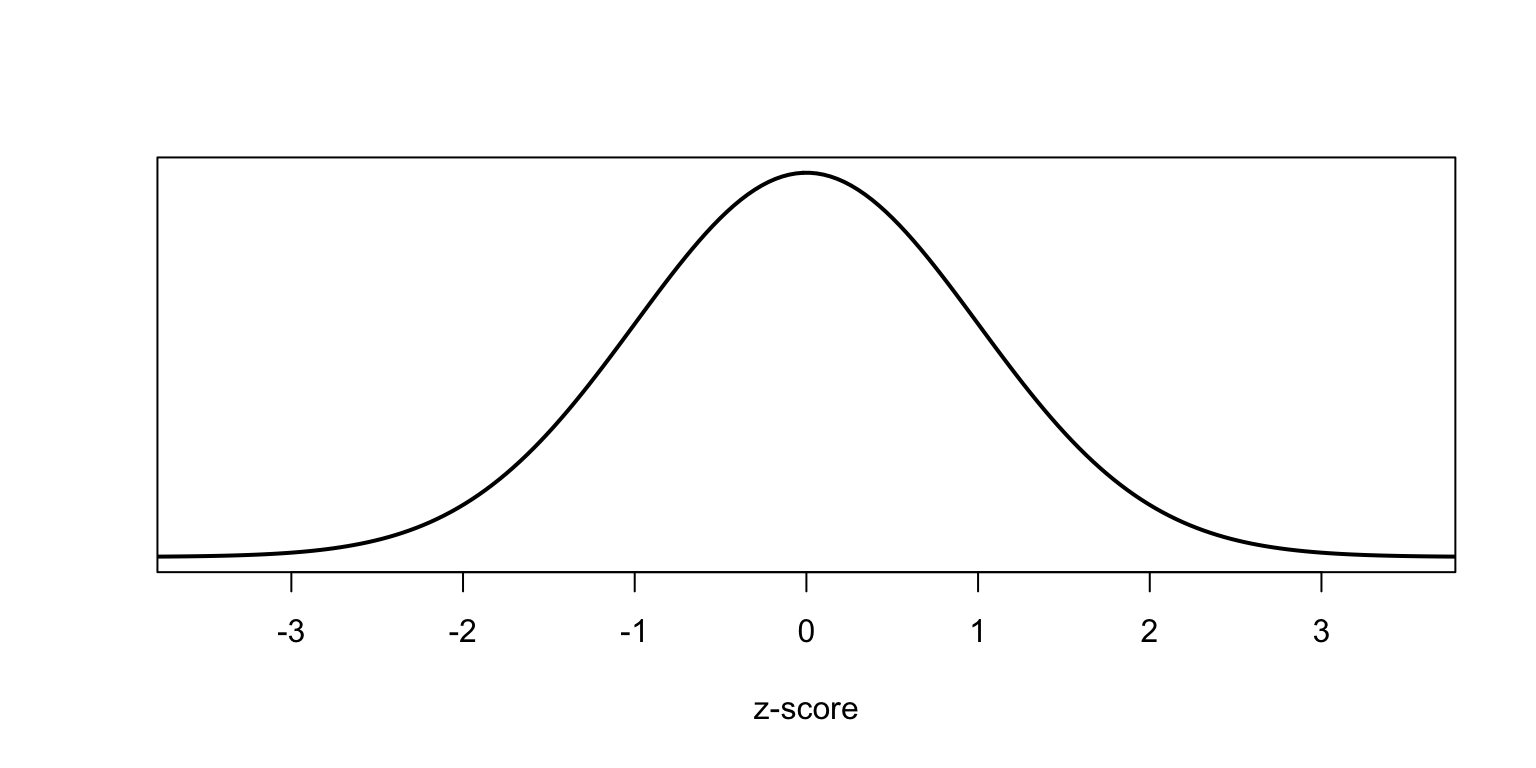## Standard Normal Distribution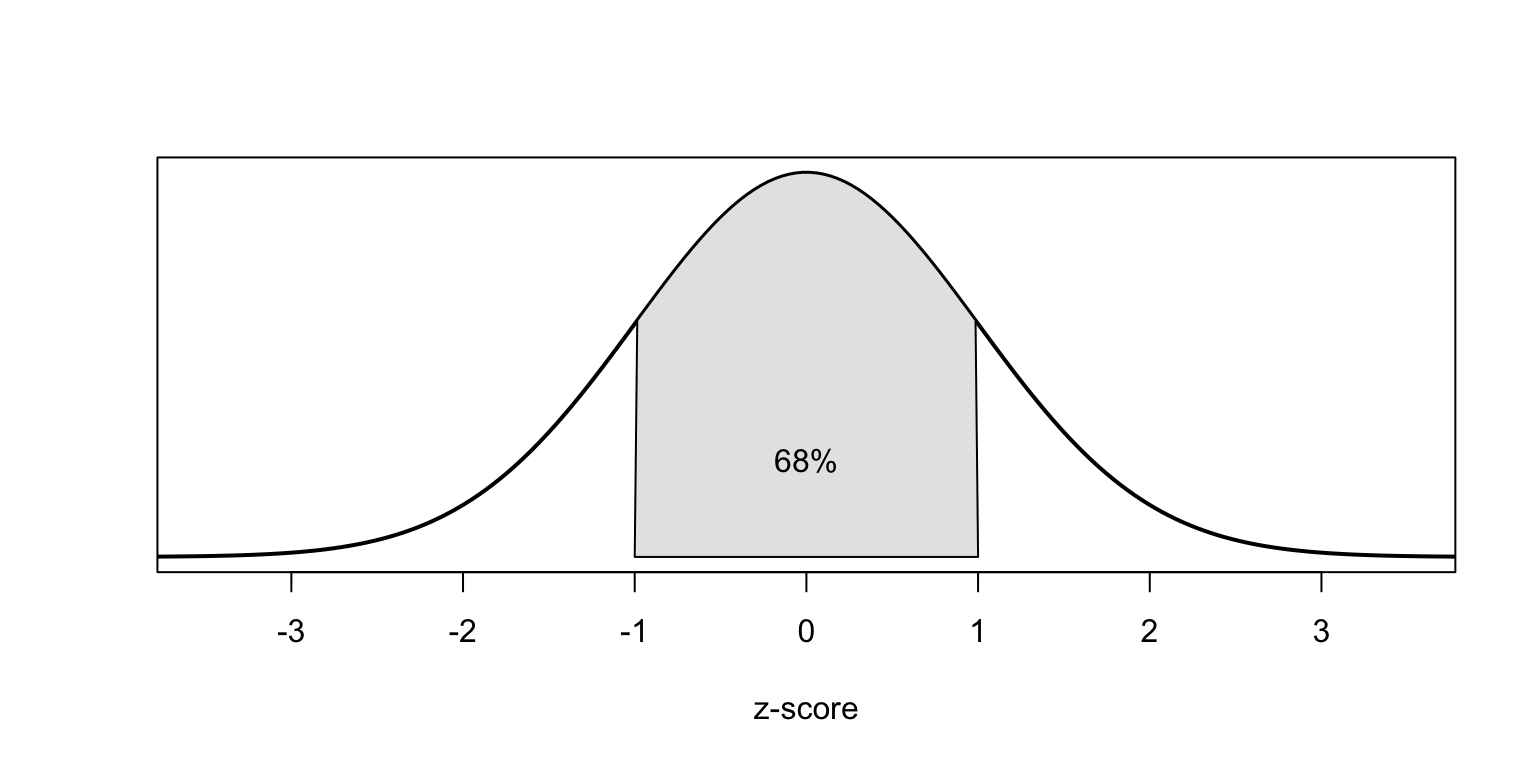## Standard Normal Distribution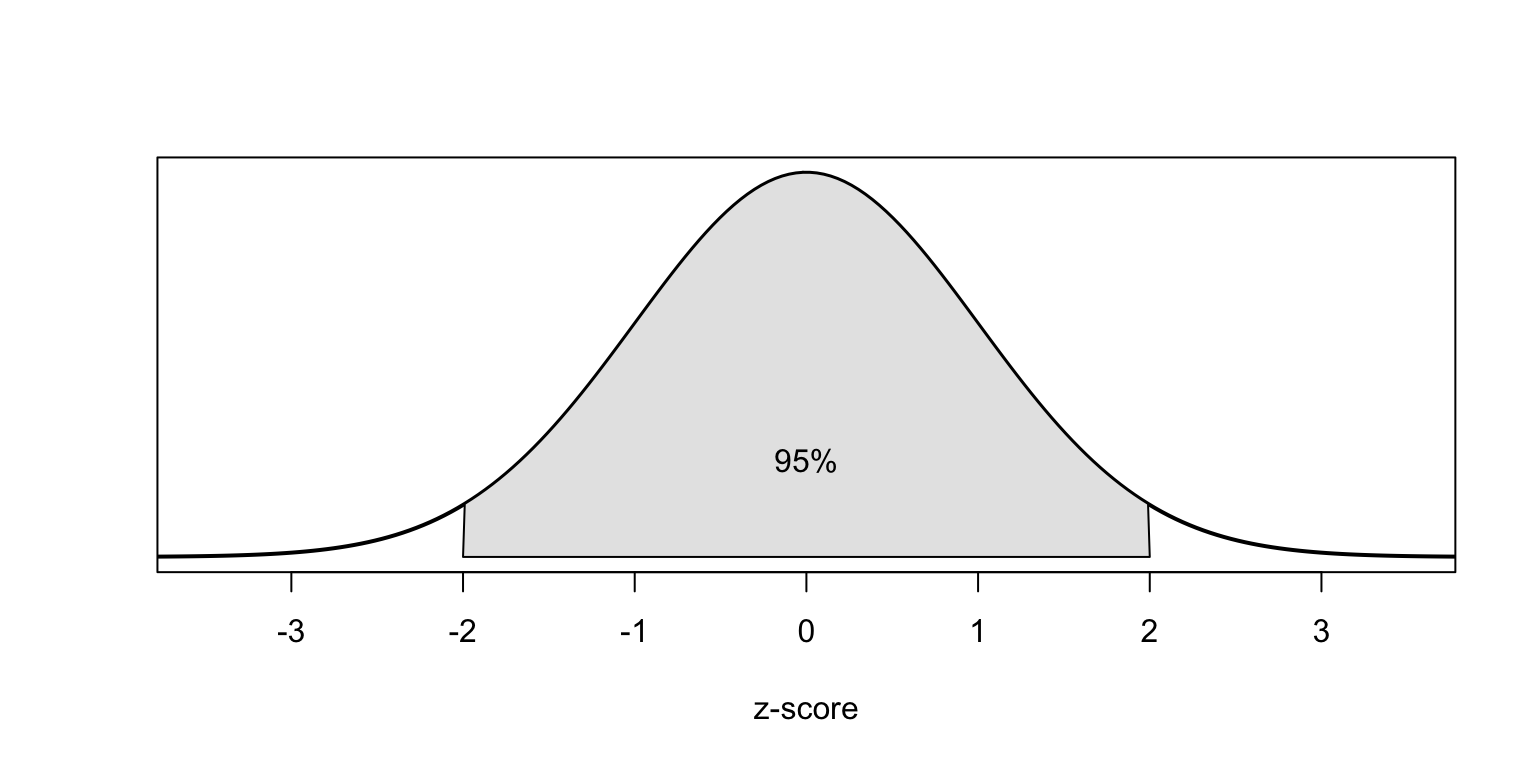## Standard Normal Distribution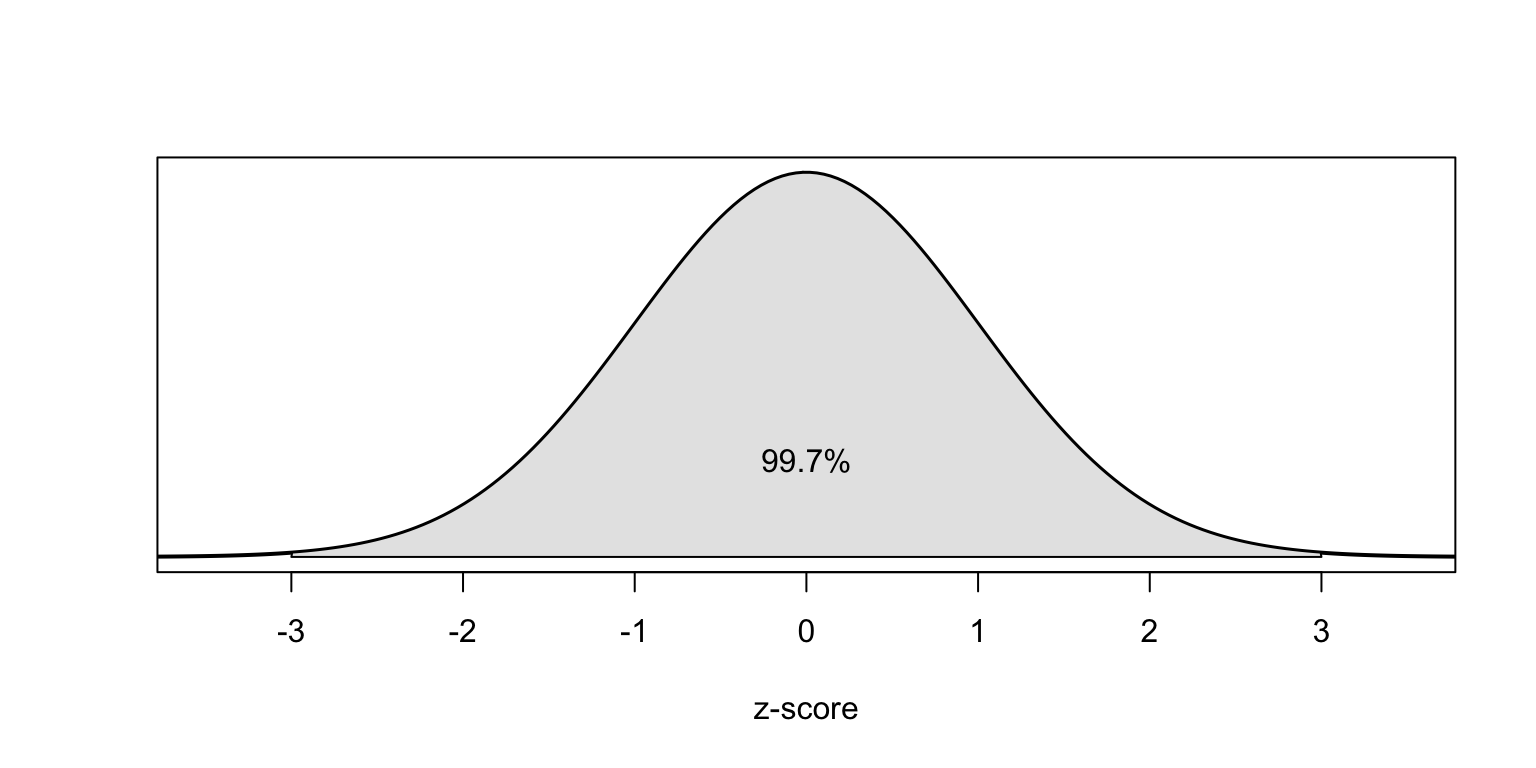## What’s the likelihood of ending with less than 15?

pnorm(15, mean=mean(samples), sd=sd(samples))
##  0.9288734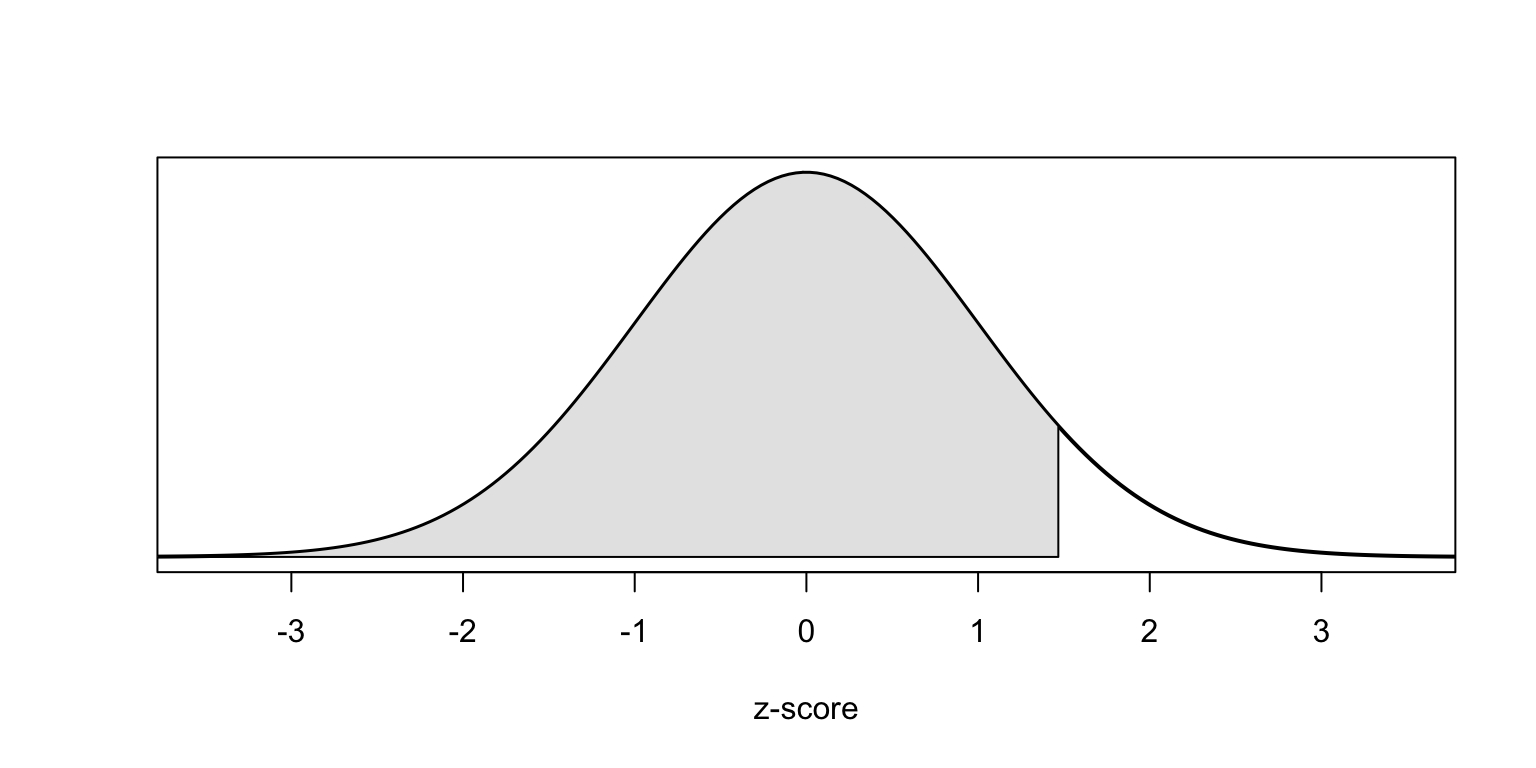## What’s the likelihood of ending with more than 15?

1 - pnorm(15, mean=mean(samples), sd=sd(samples))
##  0.07112657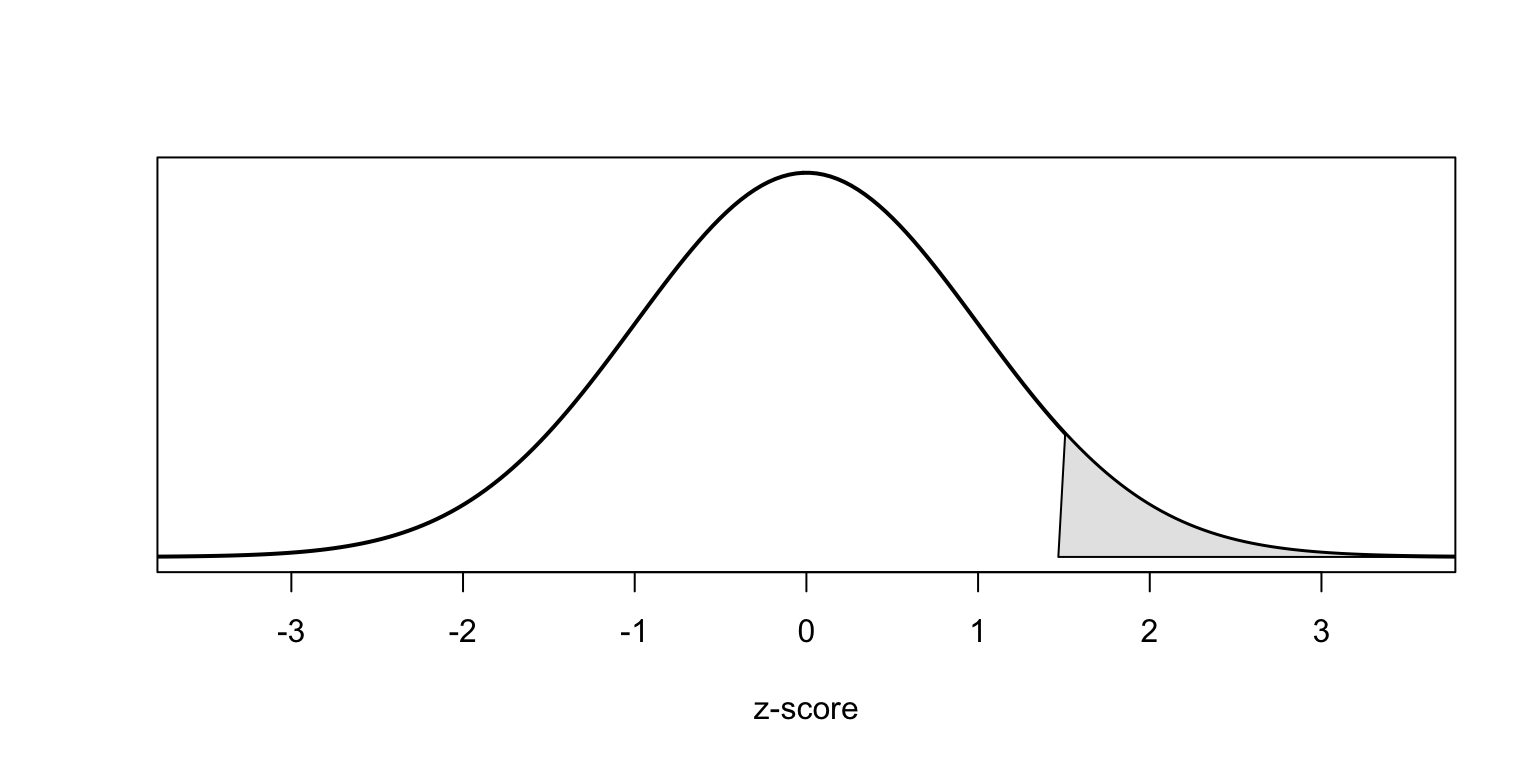## Comparing Scores on Different Scales

SAT scores are distributed nearly normally with mean 1500 and standard deviation 300. ACT scores are distributed nearly normally with mean 21 and standard deviation 5. A college admissions officer wants to determine which of the two applicants scored better on their standardized test with respect to the other test takers: Pam, who earned an 1800 on her SAT, or Jim, who scored a 24 on his ACT?

## Z-Scores

• Z-scores are often called standard scores:

$Z = \frac{observation - mean}{SD}$

• Z-Scores have a mean = 0 and standard deviation = 1.

Converting Pam and Jim’s scores to z-scores:

$Z_{Pam} = \frac{1800 - 1500}{300} = 1$

$Z_{Jim} = \frac{24-21}{5} = 0.6$